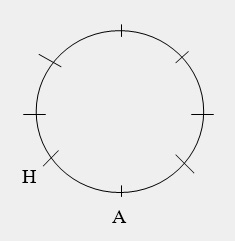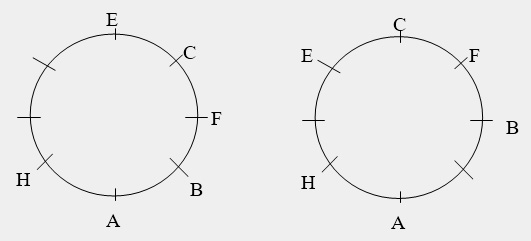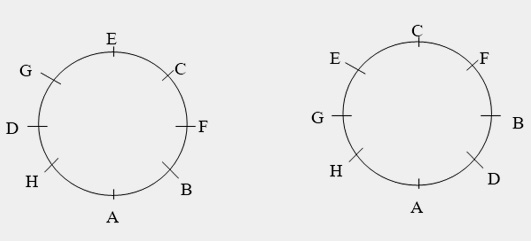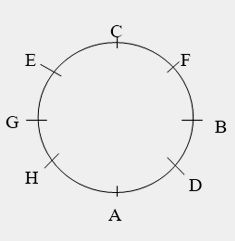# Seating Arrangement : Solved Examples

Directions for Questions 1 to 5: Read the following information carefully to answer the questions given below:
Nine persons A, B, C, D, E, F, G, H, I are watching a movie in a theatre sitting in a row facing North. B is at one end of the row. H is seated adjacent to both F and G. C is to the immediate right of D and at third place to the right of E. A is immediate left of F. F is at the third place to the left of B.
Q1. Who is sitting at the centre of the row?
1. R
2. A
3. F
4. H
Sol: Here we have to arrange 9 persons in a row. First we will fill the direct information. It is given that B is at one end of the row. So B may be at either end of the row. So we have the following two cases.
B __ __ __ __ __ __ __ __ or __ __ __ __ __ __ __ __ B
Next in the last line it is given that F is at the third place to the left of B, so the first arrangement will be rejected as there is no place to the left of B. Now fix the position of F in the second arrangement, we get
__ __ __ __ __ F__ __ B Again there is information about the position of A with respect to F. A is right next to the left of F. So if we place A at his position, the arrangement will be __ __ __ __ A F__ __ B.
Just keep on looking for the information which will relate the remaining persons with those who are already arranged. It is given that H is seated adjacent to both F and G. So H is in between  F and G. So we have __ __ __ __ A F H G B.
Now C is at third place to the right of E. So there is only one possibility that E sits at the other extreme end. We have the arrangement E __ __ C A F H G B. Now C is to the immediate right of D. So the final arrangement is E I D C A F H G B.
Hence, A is sitting at the centre of the row. Therefore, the answer is option 2.
Q2. Who is at the other end of the row?
1. A
2. E
3. G
4. C
Sol: The final arrangement is E I D C A F H G B. Hence, E is at the other end of the row. Therefore, the answer is option 2.
Q3. Which of the following statements is true?
1. E is two seats away from A.
2. D is at one extreme end.
3. I and G are neighbours.
4. There is one person between F and C
Sol: The final arrangement is E I D C A F H G B. Hence, “There is one person between F and C” is true. Therefore, answer is option 4.
Q4. What is the position of F with respect to D?
1. Second to the right
2. Second to the left
3. Third to the right
4. Fourth to the left
Sol: The final arrangement is E I D C A F H G B. Hence, F is sitting third to the right of D. Therefore, answer is option 3.
Q5. If all the friends are allowed to sit alphabetically from left to right then the position of how many friends will remain unchanged?
1. One
2. Two
3. Three
4. Position of all will change
Sol: The given arrangement is E I D C A F H G B. So if they are arranged in alphabetic order then the arrangement will be A B C D E F G H I. So the position of F will remain unchanged. Therefore, answer is option 1.
Directions for Questions 6 to 10: Read the following information and answer the given questions:
A, B, C, D, E, F, G and H are eight friends sitting round a circle facing towards the centre.
1. H is not a neighbour of E and D and he is sitting immediate left of A.
2. G is the neighbour of E. F is to the immediate right of B.
3. C is sitting between E and F.
Q6. Which of the following statements is true?
1. E is sitting between F and B
2. F is the neighbour of G
3. G is sitting between H and E
4. H is sitting between A and D
Sol: This problem is based on the circular sitting arrangement. Here the persons are sitting facing the centre. It is given that H is immediate left of A. So let us first arrange A and H.Now F is to the immediate right of B and C is sitting between E and F. It means that B, F, C and E are sitting together. Now the position of B is not defined. So we have following arrangements.Note that B cannot be third to the right of A as in that case E will become the neighbour of H which the first condition say, cannot happen. Now it is further given that G is the neighbour of E. So we can arrange, in both the above arrangements, G and D asNow in the first arrangement, D is the neighbor of H. So this is not a valid arrangement. So the final arrangement is:Hence, G is sitting between H and E is true. So, the option 3 is the answer.
Q7. What is the position of D?
1. On the immediate left of B
2. Second to the right of F
3. Between B and F
4. On the immediate left of A
Sol: According to the final arrangement.D is on the immediate left of B. So answer is option 1.
Q7. What is the position of D?
1. On the immediate left of B
2. Second to the right of F
3. Between B and F
4. On the immediate left of A
Sol: According to the final arrangement.D is on the immediate left of B. So answer is option 1.
Q8. What is the position of G?
1. Second to the left of D
2. Third of the right of F
3. On the immediate right of H
4. Between E and C
Sol: According to the final arrangement.G is third of the right of F. So answer is option 2.
Q9. Who are the neighbors of A?
1. H and D
2. H and E
3. B and C
4. E and C
Sol: According to the final arrangement.H and D are the neighbors of A. Hence the answer is option 1.
Q10. What is the position of E with respect to H?
1. Third to the left
2. Second to the right
3. Second to the left
4. Third to the right
Sol: According to the final arrangement.E is second to the left of H. Hence option 3.